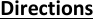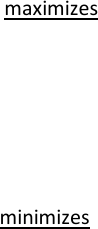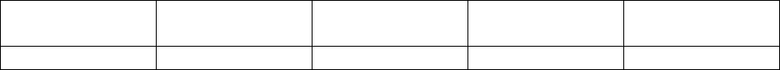# ECO 311 Miami U eco311mt1Fall 1sp18

12 views10 pages
School
Miami University
Department
Economics
Course
ECO 311
ProfessorEco311, First Midterm Exam, Spring 2018
Prof. Bill Even
1
Directions
distinguished from your work. If the logic of your work looks correct but there is a math error, you may
follow.
Round all numerical answers to the nearest 100th (e.g. 1.23) unless specifically told otherwise. Unless
indicated otherwise, each question is worth 4 points.
The formula sheet and a table with the standard normal CDF are attached to the last page of the exam.
Unlock document

This preview shows pages 1-3 of the document.
Unlock all 10 pages and 3 million more documents.Eco311, First Midterm Exam, Spring 2018
Prof. Bill Even
2
To answer the next 3 questions, consider the following relationship between annual household
spending on water, the household head’s age, and age-squared that was estimated using data from the
2016 American Community Survey.
   =258 +10.9 .10 2
1. If the household head is 30 years old, use a derivative to calculate the marginal effect of one more
year of age on water cost.
In the ACS data, heads of household range between 16 and 97 years of age.
2. What age maximizes water cost? (Your answer must be somewhere in the range of 16 to 97 years of
age).
3. What age minimizes annual water cost? (Your answer must be somewhere in the range of 16 to 97
years of age).
4. As an alternative to the linear model above, consider the following log-linear model of annual water
cost:
ln(cost of water)= 5.4 + .023age .0002age2
For a household head who is 30 years old, use a log-approximation to estimate the percent change in
water cost associated with an additional year of age. Be sure to give your answer as a percent and
Unlock document

This preview shows pages 1-3 of the document.
Unlock all 10 pages and 3 million more documents.Eco311, First Midterm Exam, Spring 2018
Prof. Bill Even
3
Consider the following alternative to the earlier equation for the cost of water that allows cost to
depend on age, the number of children living in the household (nchild.), and the interaction between
age and nchild.
   =5218 24.8 +16  11.9 ( )
5. Based on this equation for water cost, use a first order approximation to estimate the marginal
effect of an additional child on water cost for a person who is 40 years old with 2 children. Round
6. Based on the above equation, which of the following is true?
a. The marginal effect of age on water cost is more negative for families with more children.
b. The marginal effect of children on water cost is negative for all households since household
heads range in age from 16 to 97.
c. Both of the above are true
d. None of the above are true.
To answer the next 3 questions, consider the following data on the number of children living with the
household head. Those with 3 or more children are counted as having 3. You can ignore this issue in
answering the questions. I also rounded the probabilities to the nearest .05 to make calculations easier.
Number of
children
0
1
2
3
Probability
.60
.20
.15
.05
5. What is the expected number of children living with a household head?
6. What is the variance of number of children living with a household head?
7. What is [( − )3] where X is number of children living with a household head and ()=?
Unlock document

This preview shows pages 1-3 of the document.
Unlock all 10 pages and 3 million more documents.